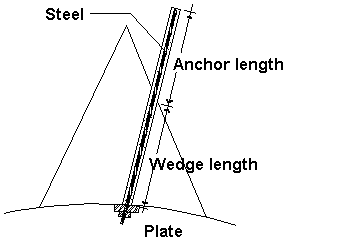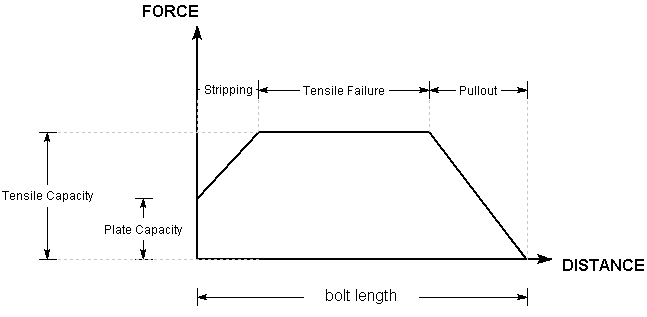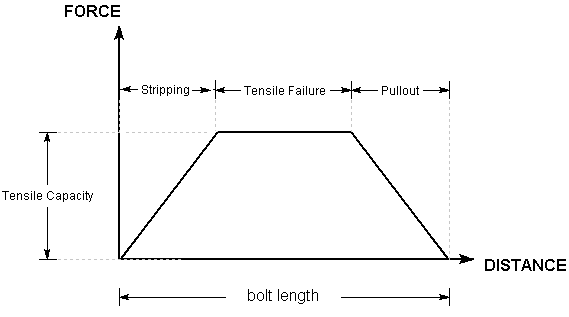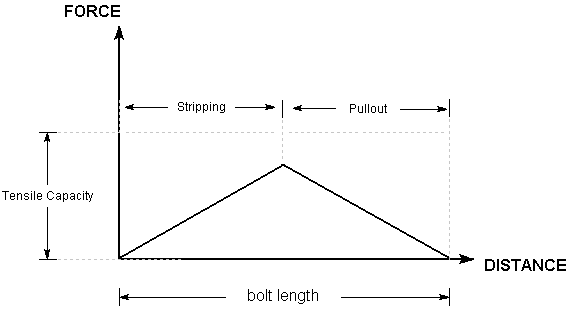# Split Set / Swellex

Split Set and Swellex bolt types in RocPlane allow you to model a fully bonded frictional bolt type, as shown in the figure below.Split Set and Swellex are presented as two different bolt types in RocPlane, for modeling convenience. However, the two bolt types are implemented in exactly the same way in the analysis. The only difference between the two selections is that the default Bond Strength is higher for Swellex than for Split Set. This value can of course be changed by the user.

Furthermore, the implementation of the Swellex / Split Set models in RocPlane is essentially the same as the Grouted Dowel model, with the condition that the Bonded Length for a Swellex / Split Set is always 100%. Except for this difference, the implementation of the Swellex / Split Set and Grouted Dowel models are the same. For details, see Grouted Dowel Bolts in RocPlane.

NOTE: To model a Swellex or Split Set bolt without a face plate, enter Plate Capacity = 0.

## Bolt Force Diagram

A Bolt Force Diagram for a Swellex bolt that exhibits all three failure modes is shown below. If the Plate Capacity is less than the Tensile Capacity, Stripping is a possible failure mode. If the Bond Capacity at either end is sufficient to equal the Tensile Capacity of the bolt, tensile failure can occur along the middle of the bolt, as shown in the following figure.Bolt Force Diagrams for Swellex or Split Set bolts with no face plate are shown below (if Plate Capacity = 0, the origin of the force diagram is at zero force). For the Swellex example, tensile failure is possible due to the relatively high bond strength of Swellex bolts. For the Split Set example, tensile failure cannot occur because the Bond Capacity is not sufficient to equal the Tensile Capacity of the bolt. Therefore, only Stripping or Pullout can occur. Actual Bolt Force Diagrams depend on Bolt Length, Bond Strength, Tensile Capacity, and Plate Capacity.Tensile bolt force diagram for Swellex bolt with no face plateTensile bolt force diagram for split set with no face plate